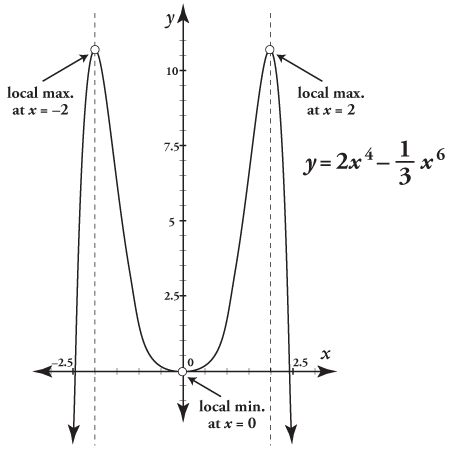##### Statistics Workbook For Dummies with Online PracticeWith the second derivative test, you use — can you guess? — the second derivative to test for local extrema. The second derivative test is based on the absolutely brilliant idea that the crest of a hill has a hump shape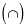and the bottom of a valley has a trough shape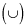After you find a function's critical numbers, you have to decide whether to use the first or the second derivative test to find the extrema. For some functions, the second derivative test is the easier of the two because

• The second derivative is usually easy to get;

• You can often plug the critical numbers into the second derivative and do a quick computation; and

• You will often get non-zero results and thus get your answers without having to do a sign graph and test regions.

The following practice questions ask you to apply the second derivative test.

## Practice questions

1. Find the local extrema of f(x) = –2x3 + 6x2 + 1 with the second derivative test.

2. Find the local extrema of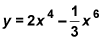with the second derivative test.

1. The local min is at (0, 1); the local max is at (2, 9).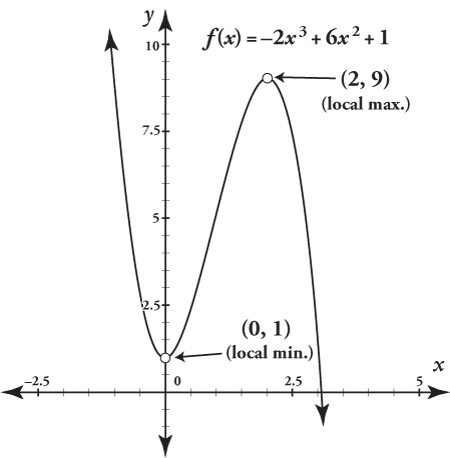You start by finding the critical numbers.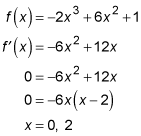Then you find the second derivative.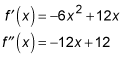Plug in the critical numbers.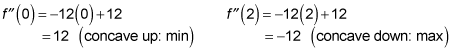Now determine the y coordinates for the extrema.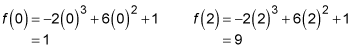So, there's a min at (0, 1) and a max at (2, 9).

2. You find local maxes at x = –2 and x = 2 with the second derivative test; you find a local min at x = 0 with street smarts.

Start by finding the critical numbers.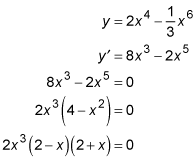So, x = 0, 2, –2.

Now get the second derivative.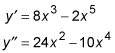Time to plug in.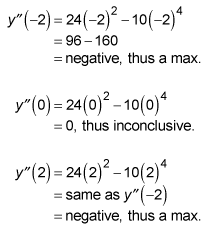Hmm. You find that the second derivative test fails at x = 0, so you have to use the first derivative test for that critical number. And this means, basically, that the second derivative test was a waste of time for this function.

Here's why: If — as in the function for this problem — one of the critical numbers is x = 0, and you can see that the second derivative will equal zero at x = 0 (because, for example, all the terms of the second derivative will be simple powers of x), then the second derivative test will fail for x = 0, and it will likely be a waste of time. You would generally be better off using the first derivative test instead.

But wait! Because this problem involves a continuous function and because there's only one critical number between the two maxes you found, the only possibility is that there's a min at x = 0. (That's where the streetwise logic comes in!)

Sure enough, here's the graph to prove it: